Module 03 (Week 3)

Thursday, May 23, 2013

10:14 AM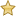Selection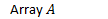• Distinct keys
• Arbitrary order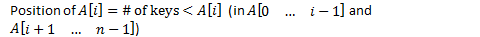•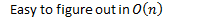Quick select

Worst Case:

• Array in ascending order
• K = n-1

Average case

• Need to consider
• All inputs of a certain size and take average
• Sum runtimes of all inputs
• Divide by # of inputs

We'll count comparisons

Assumption 1: keys are distinct

• Behaviour of algorithm on relative ordering of keys
• Not actual values
•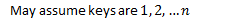•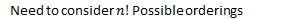Assumption 2:

• Uniform distribution
• Each permutation is equally likely
•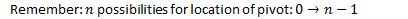•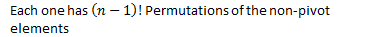• Each pivot location is equally likely
•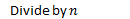After partition

Remember cases:

1.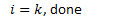1.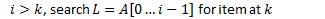1.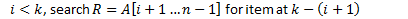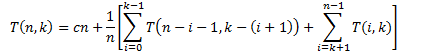Created with Microsoft OneNote 2010
One place for all your notes and information